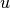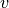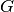Warning

This documents an unmaintained version of NetworkX. Please upgrade to a maintained version and see the current NetworkX documentation.

# Centrality¶

## Degree¶

 degree_centrality(G) Compute the degree centrality for nodes. in_degree_centrality(G) Compute the in-degree centrality for nodes. out_degree_centrality(G) Compute the out-degree centrality for nodes.

## Closeness¶

 closeness_centrality(G[, u, distance, ...]) Compute closeness centrality for nodes.

## Betweenness¶

 betweenness_centrality(G[, k, normalized, ...]) Compute the shortest-path betweenness centrality for nodes. edge_betweenness_centrality(G[, k, ...]) Compute betweenness centrality for edges.

## Current Flow Closeness¶

 current_flow_closeness_centrality(G[, ...]) Compute current-flow closeness centrality for nodes.

## Current-Flow Betweenness¶

 current_flow_betweenness_centrality(G[, ...]) Compute current-flow betweenness centrality for nodes. edge_current_flow_betweenness_centrality(G) Compute current-flow betweenness centrality for edges. approximate_current_flow_betweenness_centrality(G) Compute the approximate current-flow betweenness centrality for nodes.

## Eigenvector¶

 eigenvector_centrality(G[, max_iter, tol, ...]) Compute the eigenvector centrality for the graph G. eigenvector_centrality_numpy(G[, weight]) Compute the eigenvector centrality for the graph G. katz_centrality(G[, alpha, beta, max_iter, ...]) Compute the Katz centrality for the nodes of the graph G. katz_centrality_numpy(G[, alpha, beta, ...]) Compute the Katz centrality for the graph G.

## Communicability¶

 communicability(G) Return communicability between all pairs of nodes in G. communicability_exp(G) Return communicability between all pairs of nodes in G. communicability_centrality(G) Return communicability centrality for each node in G. communicability_centrality_exp(G) Return the communicability centrality for each node of G communicability_betweenness_centrality(G[, ...]) Return communicability betweenness for all pairs of nodes in G. estrada_index(G) Return the Estrada index of a the graph G.

 load_centrality(G[, v, cutoff, normalized, ...]) Compute load centrality for nodes. edge_load(G[, nodes, cutoff]) Compute edge load.
 dispersion(G[, u, v, normalized, alpha, b, c]) Calculate dispersion betweenandin.